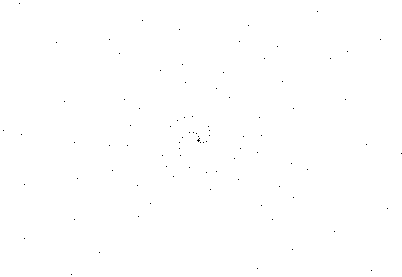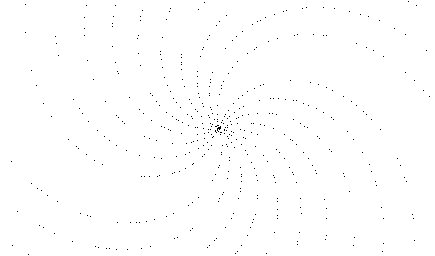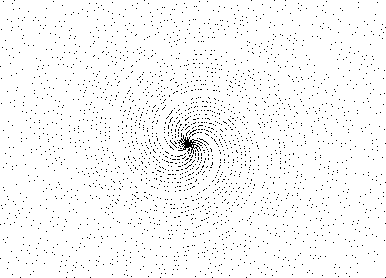# The infernal prime spiral

Let the following polar function :

 r(x)= x when x is a prime number undefined otherwise

where x is in radians

I use this UBASIC program to draw this function :

`5 cls:screen 23`

`7 input "Scale:";C`

`8 clr J`

`9 line (319,240)-(321,240):line (320,239)-(320,241)`

`10 for I=2 to 5000000`

`12 Prime=prmdiv(I)`

`13 if Prime=I inc J`

`15 if Prime=I then pset ((I*cos(I))/C+320,(I*sin(I))/C+240),1`

`17 if inkey="+" then cancel for:C=C/2:cls:goto 9`

`19 if inkey="-" then cancel for:C=C*2:cls:goto 9`

`20 next`

That's what we have at different scales (when we go away from the center) :Graph 1Graph 2Graph 3

At the beginning (Graph 1), we can only see 2 rotating arms in the spiral. But gradually (Graph 2 and 3), we can see 2 big arms in which we have exactly 10 little arms.Why 10 ? I never used this number neither in the function nor in the program. Now, if we take x in degrees, we obtain a simple Archimede spiral less and less dense (due to the prime numbers rarefaction). So, would it be an hidden property of pi ?

Thanks to :

-  Isaac Keslassy (11 Apr 1999), who has found that it wasn't a property of pi but a property of a range including pi (3.141 to 3.144) : curious, no ?

- Douglas C. Sloan (02 Oct 1999), for his philosophical explanations.

- Erick Wong (03 Oct 1999), for his excellent explanations of all the phenomenas. Here are extracts from the mail:

##### As we zoom out further, I expect to find spirals corresponding to the very good approximation 710/113. and ther should be exactly Phi(710)=280 arms.

- Alan Powell (05 Oct 1999), for his good explanations about the rotation of the graph and the number of arms.

- Serge Boisse (07 Jan 2004), who has independently discovered the "prime spiral". See http://membres.lycos.fr/boisse/maths/primes_spiral.html for more information.